#### FM442: Quantitative Methods for Finance and Risk Analysis

Jon Danielsson
Michaelmas Term 2021

## Using Black-Scholes to price an option¶

We will define a bs() function that takes a strike price, $K$, the price of the underlying asset $P$, the annual risk-free interest rate, $r$, annual volatiliry $\sigma$, and the time to maturity. This function can return the price of a European put or call option (put by default) will return the price of a European put option. We will focus only on put options in this section, but the process is analogous for call options:

In :
# Black-Scholes function
bs <- function(K, P, r, sigma, Maturity, type = "Put"){
d1 <- (log(P/K) + (r+0.5*sigma^2/2)*(Maturity))/(sigma*sqrt(Maturity))
d2 <- d1 - sigma*sqrt(Maturity)
Call <- P*pnorm(d1) - K*exp(-r*(Maturity))*pnorm(d2)
Put <- K*exp(-r*(Maturity))*pnorm(-d2) - P*pnorm(-d1)
if (type == "Put") {
return(Put)
} else if (type == "Call") {
return(Call)
} else {
return("Not a valid type")
}
}


We will work with the JP Morgan stock. Let's first load it into our environment and estimate its volatility:

In :
# Load JPM stock
y <- Y$JPM plot(y, type = "l", main = "JPM stock")We need to scale the standard deviation of the stock by$\sqrt{250}$to get the estimated yearly volatility. We use 250 because it is the number of trading days in a year (Monday - Friday). Note that this is different from the calendar days, which are 365. The latter are used when dealing with the yearly risk-free interest rate. In : # Estimating volatility with returns n <- length(y) sigma <- sd(y)*sqrt(250) sigma  0.370395546800258 In order to use the Black-Scholes formula, we need the underlying price of the asset. We will load the PRC.RData file and get the last observation available for JP Morgan: In : # Getting the last price available for JPM load("PRC.RData") P <- tail(PRC$JPM,1)
P

139.39999

We need to make assumptions on the risk-free rate and necessary parameters for an option. We will assume the risk-free rate is $r=3\%$, the maturity is 1 year, and the strike price is $K=100$:

In :
# Assuming the necessary parameters
r <- 0.03
Maturity <- 1
K <- 100


Now we can use the bs() function we defined to price the option:

In :
# Calculating the option price
put <- bs(K = K, P = P, r = r, sigma = sigma, Maturity = Maturity)
put

3.65571347437609

#### Option price as a function of the Underlying stock price¶

We have used the last price observed to calculate the price of the option.

However, we can do a plot of the option price as a function of the underlying stock price. To do so, we will create a variable called x that will contain a sequence of values between 50 and 150. You can think of this variable as the x-axis of the plot. These are all the possible underlying prices we want will consider. We will use the function seq() with a length of 1000. Then, we will call the option bs() with the variable x as the argument P, for underlying price, and the result will be a vector of length 1000, with the option price for each value in the sequence x. Finally, we plot them together:

In :
# Option price as a function of underlying stock price

# Sequence of underlying prices
x <- seq(50, 150, length = 1000)

# Plot option price
plot(x, bs(K = K, P = x, r = r, sigma = sigma, Maturity = Maturity),
type = "l", ylab = "Option", xlab = "Underlying price", lwd = 3, col = "pink", las = 1,
bty = "l", main = "Price of put option depending on underlying stock price")

# Add a point for the observed price
points(P, bs(K=K, P=P, r=r, sigma=sigma, Maturity = Maturity),
pch = 16, col="blue")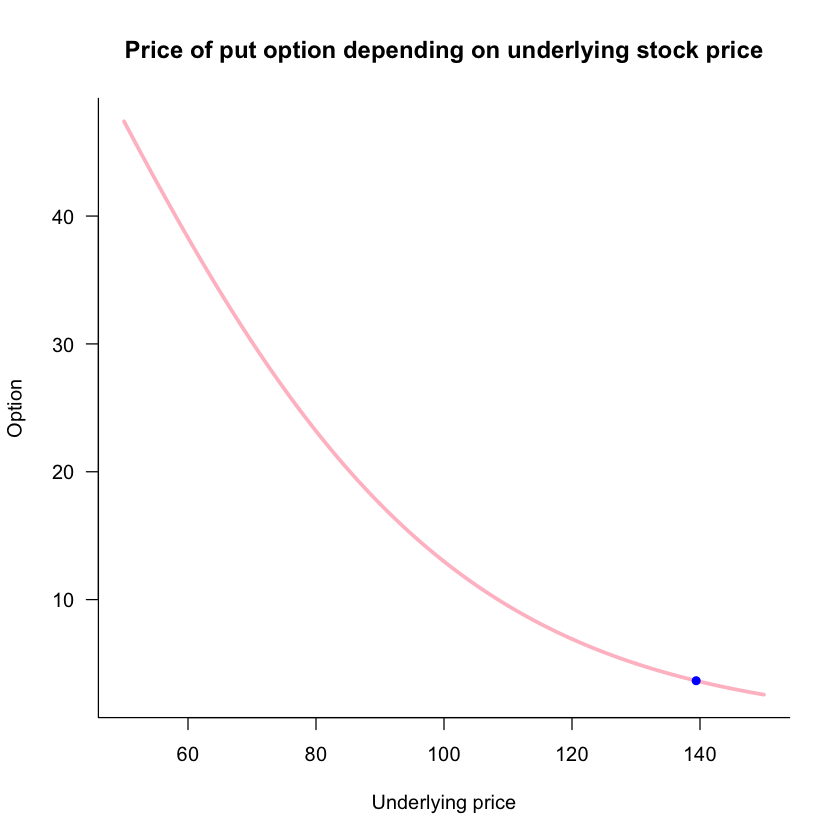We can see the put price is decreasing on the underlying price, and convex. We repeat the same procedure using a sequence for the Strike price, risk-free rate, volatility, and time to maturity:

#### Option price as a function of the Strike price¶

In :
# Option price as a function of Strike price

# Sequence of Strike
x <- seq(50, 150, length = 1000)

# Plot option price
plot(x, bs(K = x, P = P, r = r, sigma = sigma, Maturity = Maturity),
type = "l", ylab = "Option", xlab = "Strike", lwd = 3, col = "pink", las = 1,
bty = "l", main = "Price of put option depending on Strike price")

# Add a point for the observed price
points(K, bs(K=K, P=P, r=r, sigma=sigma, Maturity = Maturity),
pch = 16, col="blue")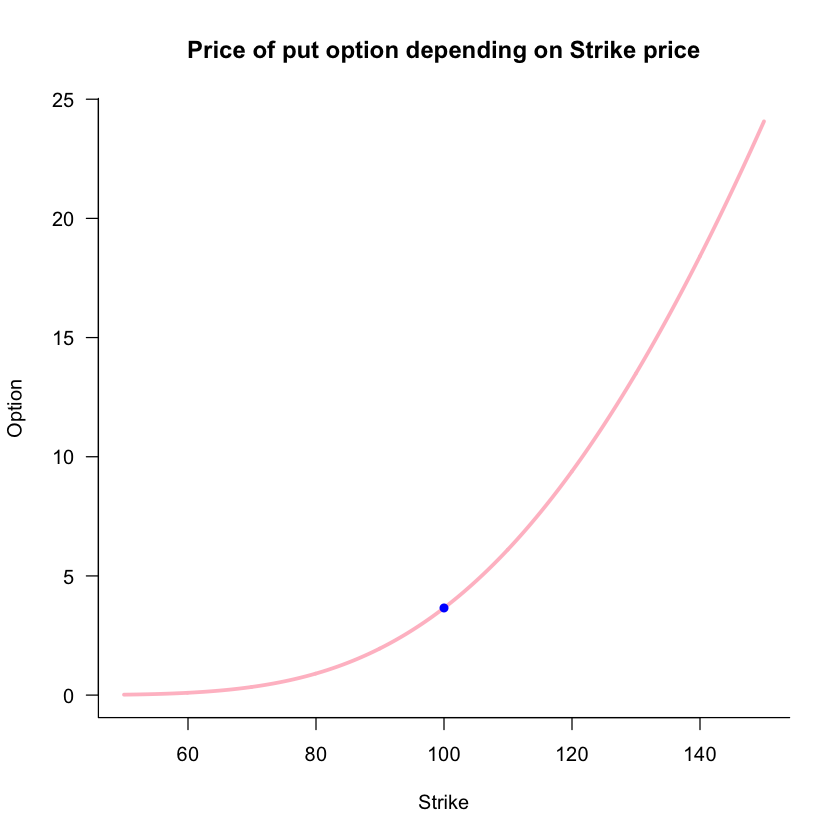The price of the put option is increasing and convex in the Strike price.

#### Option price as a function of the Risk-free rate¶

In :
# Option price as a function of risk-free rate

# Sequence of risk-free
x <- seq(0.01, 0.2, length = 1000)

# Plot option price
plot(x, bs(K = K, P = P, r = x, sigma = sigma, Maturity = Maturity),
type = "l", ylab = "Option", xlab = "Risk-free", lwd = 3, col = "pink", las = 1,
bty = "l", main = "Price of put option depending on risk-free rate")

# Add a point for the observed price
points(r, bs(K=K, P=P, r=r, sigma=sigma, Maturity = Maturity),
pch = 16, col="blue")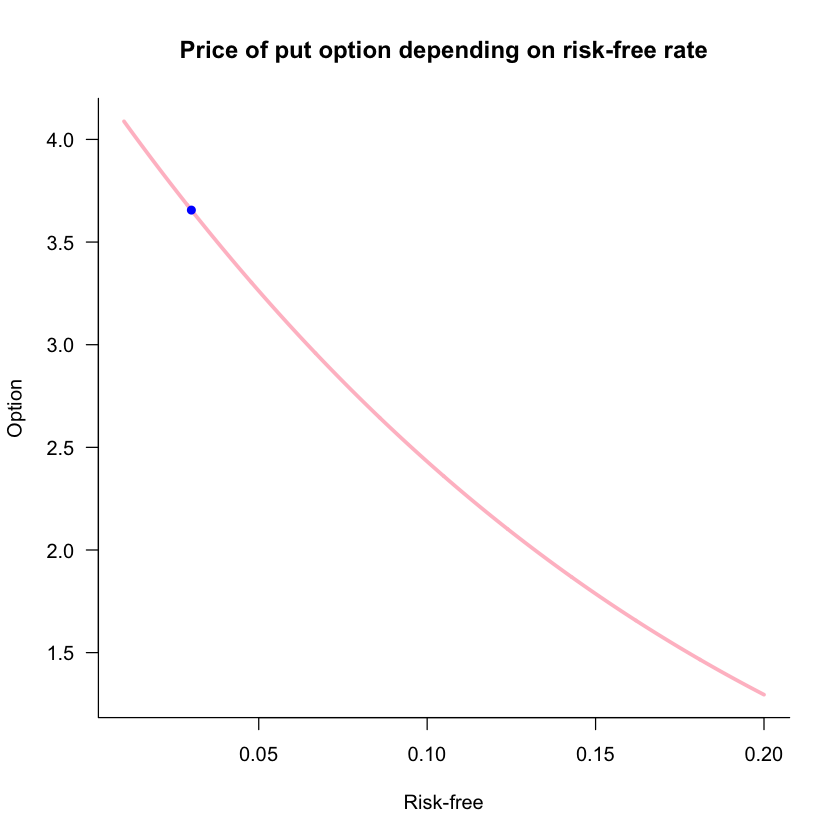The price of the put option is decreasing and convex in the risk-free rate.

#### Option price as a function of volatility¶

In :
# Option price as a function of sigma

# Sequence of sigma
x <- seq(0.01, 0.4, length = 1000)

# Plot option price
plot(x, bs(K = K, P = P, r = r, sigma = x, Maturity = Maturity),
type = "l", ylab = "Option", xlab = "Sigma", lwd = 3, col = "pink", las = 1,
bty = "l", main = "Price of put option depending on sigma")

# Add a point for the observed price
points(sigma, bs(K=K, P=P, r=r, sigma=sigma, Maturity = Maturity),
pch = 16, col="blue")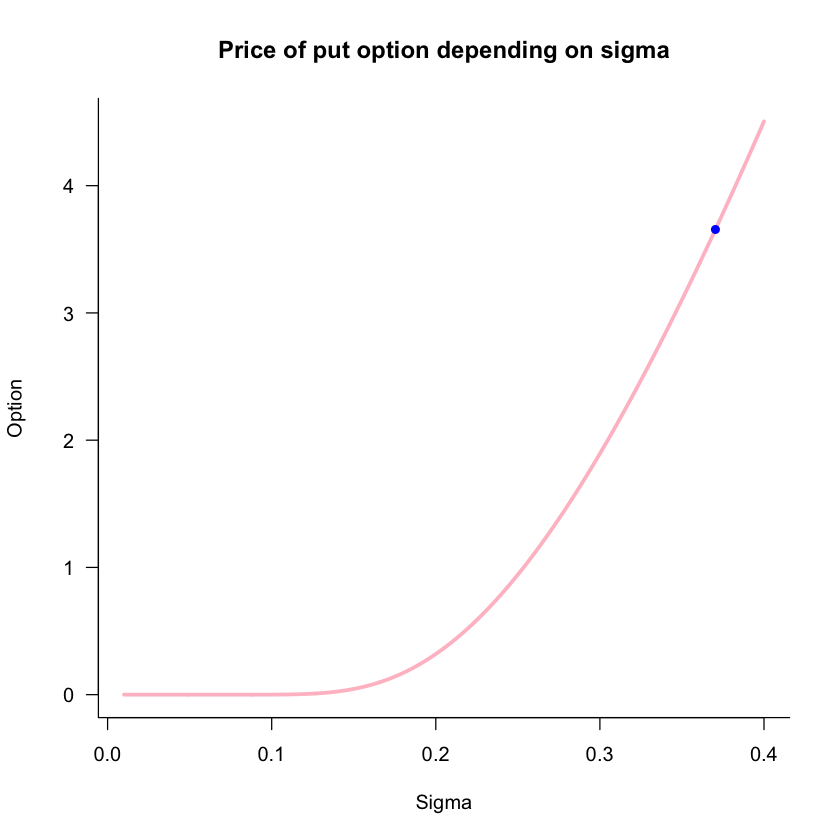The price of the option is increasing and convex in sigma, with the presence of exponential growth.

#### Option price as a function of Maturity¶

In :
# Option price as a function of Maturity

# Sequence of maturity
x <- seq(0.1, 10, length = 1000)

# Plot option price
plot(x, bs(K = K, P = P, r = r, sigma = sigma, Maturity = x),
type = "l", ylab = "Option", xlab = "Maturity", lwd = 3, col = "pink", las = 1,
bty = "l", main = "Price of put option depending on maturity")

# Add a point for the observed price
points(Maturity, bs(K=K, P=P, r=r, sigma=sigma, Maturity = Maturity),
pch = 16, col="blue")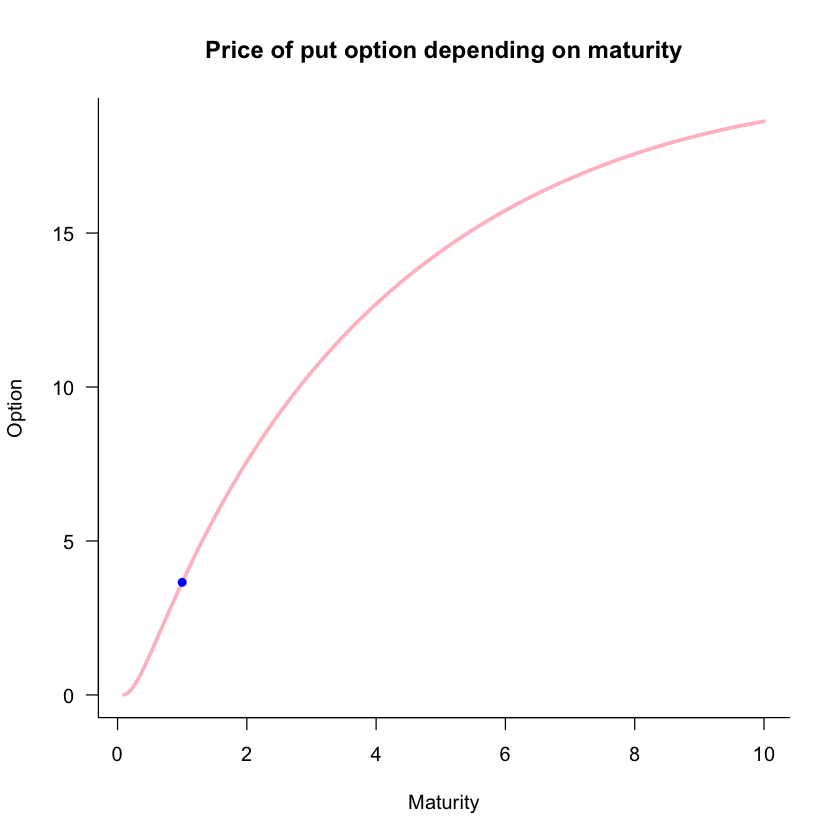The option price is increasing and concave in maturity.

## Using Monte-Carlo simulation to price an option¶

We can use a random number generator to simulate the price of an option:

In :
# Using Monte Carlo

# Simulations
S <- 1e6

# Set seed for replicability
set.seed(420)

# Get 1e6 simulations from a standard normal
ysim <- rnorm(S)

# Plot the random numbers
plot(ysim)
hist(ysim)Remember we need to apply a log-normal correction in the simulation. To do so, we subtract $\frac{1}{2}\sigma^2$ from the simulated stock returns. By default, rnorm() uses a standard normal, we would also need to scale for the desired variance:

In :
# Centering in the desired mean and changing the variance
ysim <- ysim - 0.5*sigma^2*Maturity
ysim <- ysim*sigma*sqrt(Maturity)


We can directly specify this in rnorm() to account for the variance and log-normal correction:

In :
# Specifying mean/sd in rnorm directly
set.seed(420)
ysim <- rnorm(S, mean=-0.5*sigma^2*Maturity, sd = sigma*sqrt(Maturity))

# Histogram
hist(ysim, probability = TRUE)Now we can simulate the no-arbitrage future prices of the stock:

In :
# Simulating future prices of stock
Fsim <- P*exp(r*Maturity)*exp(ysim)
hist(Fsim, probability = TRUE)To simulate the price of the put option, we subtract the simulated prices of the underlying stock from the strike price, and replace it with 0 if negative, since the option would not be executed:

In :
# Simulated price of stock
Psim <- K - Fsim

# Replacing negatives with zero
Psim[Psim<0] <- 0

# Histogram
hist(Psim, probability = TRUE)